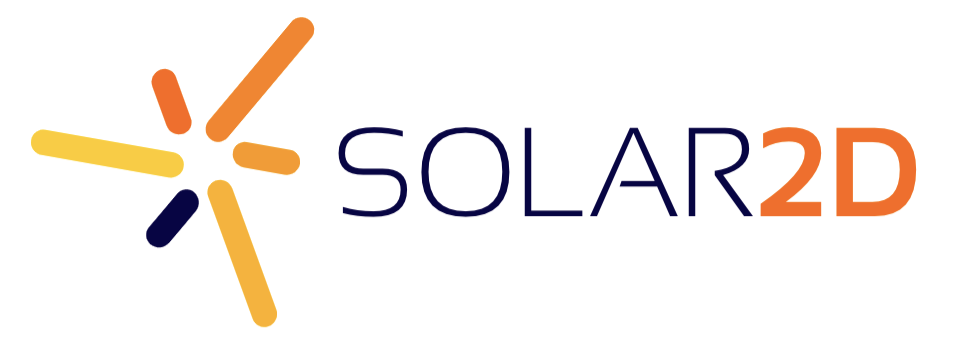# Moving objects using assigned values?

I want to set up a game where two characters rotate between 8 static spots whenever a function is called. So if character one is at position one, he moves to position number two, if he’s at two he goes to three, and so on until the eigth position where it loops. I would assign each tile a number (1-8) and the two players would also have a number matching with the tile they are standing on.

My question is if there is a command that allows me to compare the value of a character and the tiles and act accordingly. For example: Player 1 is at position number 5 and therefore has the value 5, when the function is run, his value goes up to six and therfore transitions to position number 6.

I would guess there is a way to check individual numbers for a match between two variables (that is, compare the player’s position number to the tile’s number) but I don’t know how it would be done.

Thanks in advance for any help I can get.

This will give you a solid start.  It uses a position table to coordinate the placement of tiles and movement of objects

[lua]

local cx, cy = display.contentCenterX, display.contentCenterY

local position = {}

position  = {x = cx, y = cy - 40}

position  = {x = cx + 40, y = cy}

position  = {x = cx, y = cy + 40}

position  = {x = cx - 40, y = cy}

– make tiles

local tile1 = display.newRect( position.x, position.y, 30,30 ) ; tile1.alpha = 0.4

tile1:setFillColor( 0.5, 1, 0.5 )

local tile2 = display.newRect( position.x, position.y, 30,30 ) ; tile2.alpha = 0.4

tile2:setFillColor( 0.5, 1, 0.5 )

local tile3 = display.newRect( position.x, position.y, 30,30 ) ; tile3.alpha = 0.4

tile3:setFillColor( 0.5, 1, 0.5 )

local tile4 = display.newRect( position.x, position.y, 30,30 ) ; tile4.alpha = 0.4

tile4:setFillColor( 0.5, 1, 0.5 )

– create objects

local object1 = display.newCircle( position.x, position.y, 10 )

object1:setFillColor( 1, 0.5, 0.5 )

object1.pos = 1

local object2 = display.newCircle( position.x, position.y, 10 )

object2:setFillColor( 0.5, 0.5, 1)

object2.pos = 3

local function moveObject(o)

if o.pos == 4 then

o.pos = 1

else

o.pos = o.pos + 1

end

transition.to( o, {time = 1000, x = position[o.pos].x, y = position[o.pos].y, transition = easing.outBack } )

end

local function objectsToMove()

moveObject(object1)

moveObject(object2)

end

local positionTimer = timer.performWithDelay( 2000, objectsToMove, 0)

[/lua]

Next try adding more tiles and changing their positions by adjusting the position table.  After that, add more objects and be sure you also add them to the objectsToMove( ) function.  Have fun!

Ah I see what you did here, that’s clever! I frankly was having a bit of trouble wrapping my head around tables and how they can be used. I’ll mess around with this and add more stuff now, thanks man!

You are very welcome!

Yes, tables can be very confusing at first since they can look like arrays but have much more flexibility - play around a little with the  table examples on lua.org

Basically, I used the key values , ,  etc. so that you can call coordinates from the table by referencing the position number you assign to it.  There are many ways to construct tables and this method has its limitations so be wary of adding non-number keys to the table.

This method will also work with physics if you set the linear velocity of the physics object in a direction toward the coordinates.  Of course you will have to work on the exact force to use and linear damping, and probably use counter force at some point - but it will work for physics too.

Oh I will definitely check that out, thanks! It’ll probably save me a lot of time to know how to properly use them.